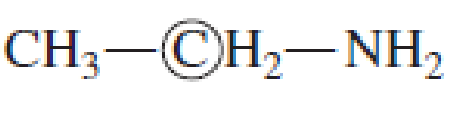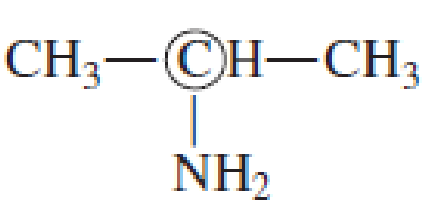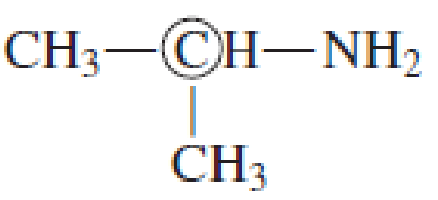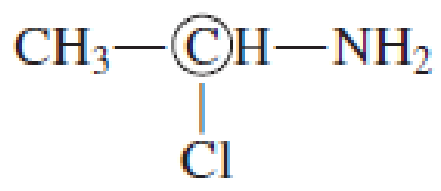Chapter 7, Problem 7.16EP### Organic And Biological Chemistry

7th Edition
STOKER + 1 other
ISBN: 9781305081079

#### Solutions

Chapter
Section### Organic And Biological Chemistry

7th Edition
STOKER + 1 other
ISBN: 9781305081079
Textbook Problem

# Indicate whether the circled carbon atom in each of the following molecules is a chiral center.(a)

Interpretation Introduction

Interpretation: The validation about the circled carbon atom in the given molecule being a chiral center has to be predicted.

Concept introduction: A center to which four different groups are attached in a tetrahedral geometry and show handedness is called chiral center.  The molecule containing the chiral center is called chiral molecule.

Explanation

Four different groups should be present in a tetrahedral arrangement, to obtain a chiral center.  The given molecule is shown below.

In the given molecule, four groups which are attached to the circled carbon are

(b)

Interpretation Introduction

Interpretation: The validation about the circled carbon atom in the given molecule being a chiral center has to be predicted.

Concept introduction: A center to which four different groups are attached in a tetrahedral geometry and show handedness is called chiral center.  The molecule containing the chiral center is called chiral molecule.

(c)

Interpretation Introduction

Interpretation: The validation about the circled carbon atom in the given molecule being a chiral center has to be predicted.

Concept introduction: A center to which four different groups are attached in a tetrahedral geometry and show handedness is called chiral center.  The molecule containing the chiral center is called chiral molecule.

(d)

Interpretation Introduction

Interpretation: The validation about the circled carbon atom in the given molecule being a chiral center has to be predicted.

Concept introduction: A center to which four different groups are attached in a tetrahedral geometry and show handedness is called chiral center.  The molecule containing the chiral center is called chiral molecule.

### Still sussing out bartleby?

Check out a sample textbook solution.

See a sample solution

#### The Solution to Your Study Problems

Bartleby provides explanations to thousands of textbook problems written by our experts, many with advanced degrees!

Get Started

#### Find more solutions based on key concepts